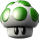5121 visningar
Quad-HullMA-cross & VWMA & MacD & TSI combination

Version 4.20

calc_on_order_fills= true, calc_on_every_tick=true, default_qty_type=strategy.percent_of_equity, default_qty_value=100, pyramiding=0
```//@version=2
//                                                    Quad-HullMA-cross & VWMA & MacD & TSI combination  <<<<< by SeaSide420 >>>>>>
strategy("MultiCross420", overlay=true, calc_on_order_fills= true, calc_on_every_tick=true, default_qty_type=strategy.percent_of_equity, default_qty_value=100, pyramiding=0)
keh=input(title="Double HullMA 1",type=integer,defval=7, minval=1)
teh=input(title="Double HullMA 2",type=integer,defval=14, minval=1)
meh=input(title="VWMA",type=integer,defval=1, minval=1)
meh1=vwma(close,round(meh))
n2ma=2*wma(close,round(keh/2))
nma=wma(close,keh)
diff=n2ma-nma,sqn=round(sqrt(keh))
n2ma1=2*wma(close,round(keh/2))
nma1=wma(close,keh)
diff1=n2ma1-nma1,sqn1=round(sqrt(keh))
n1=wma(diff,sqn)
n2=wma(diff1,sqn)
b=n1>n2?lime:red
c=n1>n2?green:red
n2ma3=2*wma(close,round(teh/2))
nma2=wma(close,teh)
diff2=n2ma3-nma2,sqn2=round(sqrt(teh))
n2ma4=2*wma(close,round(teh/2))
nma3=wma(close,teh)
diff3=n2ma4-nma3,sqn3=round(sqrt(teh))
n3=wma(diff2,sqn2)
n4=wma(diff3,sqn3)
fastLength = input(title="MacD fastLength", type=integer,defval=7)
slowlength = input(title="MacD slowlength", type=integer,defval=14)
MACDLength = input(title="MacD Length", type=integer,defval=3)
MACD = ema(close, fastLength) - ema(close, slowlength)
aMACD = ema(MACD, MACDLength)
delta = MACD - aMACD
a1=plot(n1,color=c),a2=plot(n2,color=c)
plot(cross(n1, n2) ? n1 : na, style = cross, color=b, linewidth = 3)
a3=plot(n3,color=c),a4=plot(n4,color=c)
plot(cross(n3, n4) ? n1 : na, style = cross, color=b, linewidth = 3)
//a5=plot(meh1,color=c)
long = input(title="TSI Long Length", type=integer, defval=5)
short = input(title="TSI Short Length", type=integer, defval=3)
signal = input(title="TSI Signal Length", type=integer, defval=2)
linebuy = input(title="TSI Upper Line", type=integer, defval=4)
linesell = input(title="TSI Lower Line", type=integer, defval=-4)
price = close
double_smooth(src, long, short) =>
fist_smooth = ema(src, long)
ema(fist_smooth, short)
pc = change(price)
double_smoothed_pc = double_smooth(pc, long, short)
double_smoothed_abs_pc = double_smooth(abs(pc), long, short)
tsi_value = 100 * (double_smoothed_pc / double_smoothed_abs_pc)
closelong = n1<n2 and n3<n4 and n1>meh1
if (closelong)
strategy.close("Long")
closeshort = n1>n2 and n3>n4 and n1<meh1
if (closeshort)
strategy.close("Short")
longCondition = strategy.opentrades<1 and n1>n2 and MACD>aMACD and n1<meh1 and n3>n4 and ema(tsi_value, signal)>linesell
if (longCondition)
strategy.entry("Long",strategy.long)
shortCondition = strategy.opentrades<1  and n1<n2 and MACD<aMACD and n1>meh1 and n3<n4 and ema(tsi_value, signal)<linebuy
if (shortCondition)
strategy.entry("Short",strategy.short)```

## KommentarerAllMountain
@AllMountain, yes.
it needs to be edited.
everywhere it says ""close"", change to a ""ohlc4""
SvaraSeaSide420
@SeaSide420, ohlc4 but change the things to brackets. this website not allow to type brackets
SvaraSeaSide420
@SeaSide420,
Hi,
I do not understand what you said.
Could you post a picture of the code of "close -> ohlc4"?
I would appreciate if you can.
Svara